# Place Value, Rounding, Addition, and Subtraction

Students learn to compare numbers, round to any place value, work towards fluency with the standard algorithms for adding and subtracting, and solve multi-step word problems involving addition and subtraction.

Math

Unit 1

## Unit Summary

In the first unit for Grade 4, students extend their work with whole numbers and use this generalized understanding of the place value system in the context of comparing numbers, rounding them, and adding and subtracting them.

Students understanding of the base ten system begins as early as Kindergarten, when students learn to decompose teen numbers as ten ones and some ones (K.NBT.1). This understanding continues to develop in Grade 1, when students learn that ten is a unit and therefore decompose teen numbers into one ten (as opposed to ten ones) and some ones and learn that the decade numbers can be referred to as some tens (1.NBT.2). Students also start to compare two-digit numbers (1.NBT.3) and add and subtract within 100 based on place value (1.NBT.4—6). In second grade, students generalize the place value system even further, understanding one hundred as a unit (2.NBT.1) and counting, reading, writing, comparing, adding, and subtracting numbers within 1,000 (2.NBT.2—9). In Grade 3, place value standards are additional cluster content, but they still spend time fluently adding and subtracting within 1,000 and rounding three-digit numbers to the nearest 10 and 100 (3.NBT.1—2).

Thus, because students did not focus heavily on place value in Grade 3, Unit 1 begins with where things left off in Grade 2 of understanding numbers within 1,000. Students get a sense of the magnitude of each place value by visually representing the place values they are already familiar with and building from there. Once students have a visual and conceptual sense of the “ten times greater” relationship between places, they are able to articulate why a digit in any place represents 10 times as much as it represents in the place to its right (4.NBT.1). Next, students write multi-digit numbers in various forms and compare them (4.NBT.2). Then, students learn to round whole numbers to any place value (4.NBT.3). Next, students add and subtract multi-digit whole numbers using the standard algorithms (4.NBT.4) and apply their algorithmic knowledge to solve word problems. The unit culminates with multi-step word problems involving addition and subtraction, using a letter to represent the unknown quantity, then using rounding to assess the reasonableness of their answer (4.OA.3), allowing for students to connect content across different clusters and domains (4.NBT.A, 4.NBT.B, and 4.OA.A).

Throughout the unit, students will repeatedly look for and make use of structure, specifically the structure of the place value system (MP.7). Students develop an understanding that a digit in any place represents 10 times as much as it represents in the place to its right, then apply that understanding to compare, round, and add and subtract multi-digit whole numbers.

In subsequent grade levels, students generalize their base ten understanding to decimals. While students do some work with tenths and hundredths later on in Grade 4 (4.NF.5—7), students in Grade 5 are able to extend the decimal system to many more place values, seeing that a digit represents $$\frac{1}{10}$$ of what it represents in the place to its left (5.NBT.1—3). Students subsequently round, compare, and operate on decimals as they did with whole numbers in Grade 4. Thus, this unit sets a precedent for a deep understanding of the number system that supports much of their mathematical knowledge later this year and in years to come.

Fishtank Plus for Math

Unlock features to optimize your prep time, plan engaging lessons, and monitor student progress.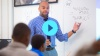## Assessment

This assessment accompanies Unit 1 and should be given on the suggested assessment day or after completing the unit.

## Unit Prep

### Intellectual Prep

Unit Launch

To learn more about how to prepare a unit, internalize a lesson, and understand the different components of a Fishtank ELA lesson, visit our Preparing to Teach Fishtank ELA Teacher Tool.#### Intellectual Prep for All Units

• Read and annotate “Unit Summary” and “Essential Understandings” portion of the unit plan.
• Do all the Target Tasks and annotate them with the “Unit Summary” and “Essential Understandings” in mind.
• Take the Post-Unit Assessment.

#### Unit-Specific Intellectual Prep

• Read the article, "Modeling With Mathematics" from Teaching Channel and watch the first video about Three-Act Tasks.
• Read the following table that includes models used in this unit.
 Concrete or pictorial base ten blocks Example: Represent 1,342 with base ten blocks.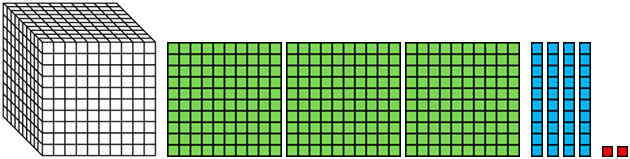Number line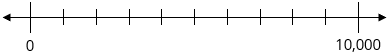Standard algorithm for addition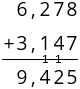Standard algorithm for subtraction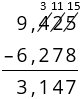Tape diagram Example: A grocery store sells 1,724 red apples and 862 green apples. How many apples did the grocery store sell?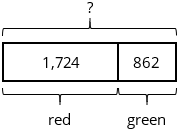### Essential Understandings

• Our place value system is structured such that a digit in any place represents 10 times as much as it represents in the place to its right.
• “To read numerals between 1,000 and 1,000,000, students need to understand the role of commas. Each sequence of three digits made by commas is read as hundreds, tens, and ones, followed by the name of the appropriate base-thousand unit (thousand, million, billion, trillion, etc.)” (NBT Progression, p. 13).
• Comparing numbers written in standard form uses the understanding that 1 of any unit is greater than any amount of a smaller unit. Thus, the largest place values in each number contains the most relevant information when comparing numbers. If both numbers have the same number of largest units, the next largest place value should be attended to next, iteratively until one digit is larger than another in the same unit.
• When rounding a number, the goal is to approximate the number by the closest number with no units of smaller value (e.g., so 4,563 to the nearest ten is 4,560; to the nearest hundred is 4,600; and to the nearest thousand is 5,000). When a number that is being rounded has a 5 in the place being considered and 0 in all smaller places, it is equidistant from the two benchmarks. Therefore, it is simply a convention that the number is rounded to the greater benchmark.
• The standard algorithms for addition and subtraction are based in the idea of needing to add like-units together and the idea that one can regroup 1 of any unit to be 10 of the next smallest unit (and vice versa).
• Rounding numbers can help one to determine whether an answer is reasonable, based on whether the estimate is close to the computed answer or not.
• Making sense of problems and persevering in solving them is an important practice when solving word problems. Key words do not always indicate the correct operation.

### Vocabulary

ten thousand

million

hundred thousand

To see all the vocabulary for Unit 1, view our 4th Grade Vocabulary Glossary.

### Materials

• Blank hundreds chart (10 per student)

• Stapler (1 or more per teacher)

• Random number generator (1 per teacher) — This can be a ten-sided die, a spinner of digits 0-9, Digit Cards for 0-9, or an online random number generator.

• Thousands Place Value Chart (Total of 3 per student) — Students might need more or less depending on their reliance on this tool.

• Base ten blocks (Maximum of 5 thousands, 40 hundreds, 50 tens, and 30 ones per student or small group) — Students might not need this many depending on their reliance on concrete materials. You could just use one set for the teacher if materials are limited.

• paper hundreds flats (4 count) (At least 25 per class period)

• Millions Place Value Chart (Total of 14 per student) — Students might need more or less depending on their reliance on this tool.

• Tape or stapler (1 or more per teacher)

## Unit Practice

Word Problems and Fluency Activities

Access daily word problem practice and our content-aligned fluency activities created to help students strengthen their application and fluency skills.## Lesson Map

Topic A: Place Value of Multi-Digit Whole Numbers

Topic B: Reading, Writing, and Comparing Multi-Digit Whole Numbers

Topic C: Rounding Multi-Digit Whole Numbers

Topic D: Multi-Digit Whole-Number Addition and Subtraction

## Common Core Standards

Key

Major Cluster

Supporting Cluster

### Core Standards

#### Number and Operations in Base Ten

• 4.NBT.A.1 — Recognize that in a multi-digit whole number, a digit in one place represents ten times what it represents in the place to its right. For example, recognize that 700 ÷ 70 = 10 by applying concepts of place value and division.
• 4.NBT.A.2 — Read and write multi-digit whole numbers using base-ten numerals, number names, and expanded form. Compare two multi-digit numbers based on meanings of the digits in each place, using >, =, and < symbols to record the results of comparisons.
• 4.NBT.A.3 — Use place value understanding to round multi-digit whole numbers to any place.
• 4.NBT.B.4 — Fluently add and subtract multi-digit whole numbers using the standard algorithm.

#### Operations and Algebraic Thinking

• 4.OA.A.3 — Solve multistep word problems posed with whole numbers and having whole-number answers using the four operations, including problems in which remainders must be interpreted. Represent these problems using equations with a letter standing for the unknown quantity. Assess the reasonableness of answers using mental computation and estimation strategies including rounding.

• 2.MD.B.6

• 2.NBT.A.1
• 2.NBT.A.2
• 2.NBT.A.3
• 2.NBT.A.4
• 3.NBT.A.1
• 3.NBT.A.2
• 3.NBT.A.3

• 3.OA.D.8

• 5.NBT.A.1
• 5.NBT.A.2
• 5.NBT.A.3
• 5.NBT.A.4
• 5.NBT.B.6
• 5.NBT.B.7

### Standards for Mathematical Practice

• CCSS.MATH.PRACTICE.MP1 — Make sense of problems and persevere in solving them.

• CCSS.MATH.PRACTICE.MP2 — Reason abstractly and quantitatively.

• CCSS.MATH.PRACTICE.MP3 — Construct viable arguments and critique the reasoning of others.

• CCSS.MATH.PRACTICE.MP4 — Model with mathematics.

• CCSS.MATH.PRACTICE.MP5 — Use appropriate tools strategically.

• CCSS.MATH.PRACTICE.MP6 — Attend to precision.

• CCSS.MATH.PRACTICE.MP7 — Look for and make use of structure.

• CCSS.MATH.PRACTICE.MP8 — Look for and express regularity in repeated reasoning.

Unit 2

Multi-Digit Multiplication